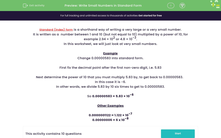# Write Small Numbers in Standard Form

In this worksheet, students will read and write small numbers in standard index form.Key stage:  KS 3

Curriculum topic:   Number

Curriculum subtopic:   Interpret/Compare Standard Form Numbers

Difficulty level:#### Worksheet Overview

Standard index form is a shorthand way of writing a very large or a very small number.

It is written as a  number between 1 and 10 (but not equal to 10) multiplied by a power of 10, for example 2.94 × 102 or 4.8 × 10-3.

You can probably see how much easier it is to manage numbers when they are in standard form - counting all those zeros can be quite tricky! It can be incredibly useful in science if you want to compare the magnitude of things that are very large (such as distances in space) or very small (such as the size of bacteria or cells in the body).In this activity, we will just look at very small numbers.

Example

Change 0.00000583 into standard form.

First, fix the decimal point after the first non-zero digit to get a number between 1 and 10:  5.83

Next, determine the power of 10 that you must multiply 5.83 by, to get back to 0.00000583

In this case it is -6.

In other words, we divide 5.83 by 10 six times to get to 0.00000583.

So 0.00000583 = 5.83 × 10-6

Other Examples

0.0000001122 = 1.122 × 10-7

0.00000006 = 6 x 10-8

Let's try some questions now.

### What is EdPlace?

We're your National Curriculum aligned online education content provider helping each child succeed in English, maths and science from year 1 to GCSE. With an EdPlace account you’ll be able to track and measure progress, helping each child achieve their best. We build confidence and attainment by personalising each child’s learning at a level that suits them.

Get started••••